Deutsche VersionConverting number relations (ratio) to dB level
● number - factor - ratio - parameter ●

Voltage ratio: Level L (dB) = 20 × log (value 2 / value 1)   That are field quantities
Power ratio: Level L (dB) = 10 × log (value 2 / value 1)   That are energy quantities

 Output value Input value   Reference value Level L   dB

Relative level - dB chart (decibel) - volt, watt and pascal, W/m2

 Power is like all energy quantities primarily a calculated value.

 To use the calculator, simply enter a value. The calculator works in both directions of the ↔ sign.

Field quantity (size) − Voltage

 Voltage V (audio)   Volt ↔ Voltage level LV (audio)  dBu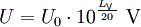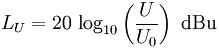Reference voltageV0 = 0.7746 volt ≡ 0 dBu
 Voltage V   Volt ↔ Voltage level LV dBV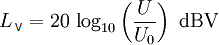Reference voltage V0 = 1 volt ≡ 0 dBV

Energy quantity (size) − Power

 Electric power P   Watt ↔ Electric power level LP dB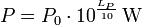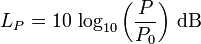Reference power P0 = 1 watt = 1 W ≡ 0 dB

There is also the reference power P0 = 1 milliwatt = 0,001 watt ≡ 0 dBm

 Electric power (telephone) P   Watt ↔ Electric power level LP dBmReference powerP0 = 1 milliwatt = 0.001 watt ≡ 0 dBm
 Another difficult issue that has to do with sound field sizes and energy sizes is: How much does the volume (loudness) decrease with the distance from a sound source? How much decreases the sound pressure (voltage) with the distance from a sound source? How much decreases the sound intensity (not the sound power) with the distance from a sound source? The initial question is quite simply this: How much does the sound decrease with distance?

 Sound power or sound power level has nothing (!) to do with the distance from the sound source. Thinking helps: A 100 watt light bulb has in 1 m and in 10 m distance really always the same 100 watts, which is emitted from the lamp all the time. Watts don't change with distance.   A frequent question: "Does the sound power depend on distance?" The clear answer is: "No, not really."

 Acousticians and sound protectors ("noise fighters") need the sound intensity (acoustic intensity) – but sound engineers and sound designers ("ear people") don't need that sound energy quantity.   Who is involved in audio engineering, should rather take care of the sound field quantity, that is the sound pressure or the sound pressure level (SPL) as an effect at the eardrums of our hearing and on the membranes of the microphones, and the corresponding audio voltage and its voltage level.